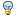# Open Top Track

If the rotor is exposed to high altitude air at 300K and ambient pressure, the air molecules will collide with the rotor surface and leave rotor velocity (WAG). They will collide with other molecules and heat them, creating a moving current of hot thin air that follows the rotor. Because they are very hot, maintaining ambient pressure with the surrounding air is accomplished at much lower density.

Assuming 120 km altitude: 360K, 2.6 mPa, 2.2e-8 kg/m3, N2 atmosphere. Molecular weight is 28, or 28×1.66e-27 = 4.65e-26 kg. 1.5kT = 1.5×360×1.38e-23 = 7.5e-21 J, so the thermal velocity V;;th;; = sqrt(2 * 7.5e-21 / 4.65e-26) = 570 m/s . Heated, ionized nitrogen with an atomic mass of 2.32e-26 kg and a thermal velocity V;;th;; of 14,000 m/s (!!!) has an energy of 2.3e-18 J and a "temperature" of 165,000 Kelvinsand so is 290 times less dense as ambient air at the same pressure, about 1e-10 kg/m3 .

What is the "skin drag power" on the rotor at this pressure?

Delivery rate of atoms, density (kg/m3) and perpendicular velocity 1/2 kT ? (m/s) -> mass rate kg/m2-s . "Friction" = power loss per unit area, = 0.5 * mass rate * rotorv2 = kg / s3 = kg m2/s2 / s -m2.

• Density = 1e-10 kg/m3
• Perpendicular velocity = 14000/sqrt(3) m/s
• Power loss per unit area = 1e-9×14,0003/sqrt(12) = 80 W/m2

Rotor area for 2000 km loop track = 80×0.16×2e6 m2 = 26 MW ... tolerable.

It might be smaller - the entrained gas is moving at some fraction of rotor velocity, and the mean free path is 3.3 meters for the ambient air. The bouncing atoms may travel quite a distance before thermalizing. OTOH, the incoming atoms might not hit any hot atoms, either. More to ponder ...

OpenTop (last edited 2016-12-13 20:41:46 by KeithLofstrom)